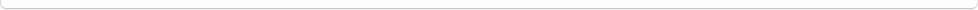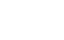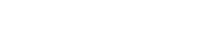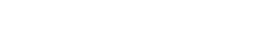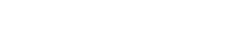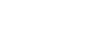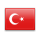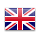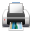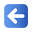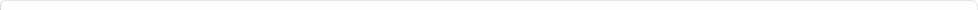Description of Individual Course Units
 Course Unit Code Course Unit Title Type of Course Unit Year of Study Semester Number of ECTS Credits 180102004100 PROBABILITY AND STATISTICS Compulsory 1 2 4
Level of Course Unit
First Cycle
Objectives of the Course
To solve problems related to civil engineering with applied statistics science
Name of Lecturer(s)
Prof. Dr. Ecevit EYDURAN
Learning Outcomes
 1 Can be able to explain the basic concepts of statistics 2 Can be able to explain the basic concepts of statistics 3 Can be able to edit the compiled data and graphically display it 4 Can be able to edit the compiled data and graphically display it 5 Can be able to calculate and explain mean, variability and dispersion measures 6 Can be able to calculate and explain mean, variability and dispersion measures 7 Can be able to make basic statistical calculations 8 Can be able to make basic statistical calculations
Mode of Delivery
Daytime Class
Prerequisites and co-requisities
None
Recommended Optional Programme Components
None
Course Contents
Definition of statistic, Collection and arrangement of data, Measures of central tendency, Measures of distribution, skewness and kurtosis, Probability, Sampling distributions, Hypothesis tests, Regression analysis, Analysis of variance.
Weekly Detailed Course Contents
 Week Theoretical Practice Laboratory 1 Introduction (Definition of Statistic, Purpose of Statistic, Some Basic Concepts) 2 Data collection and arrangement (the collection of data, organizing data, arranged in a series of statistical data, graphical presentation of the data) 3 Measures of central tendency (Arithmetic, geometric and harmonic mean, median, mode (peak value), properties of measurements and comparisons) 4 Distribution, slope and kurtosis measures (change interval, coefficient of variation, standard deviation, skewness coefficient, kurtosis coefficient) 5 Probability (Concept of probability, Basic probability rules, Conditional probability) 6 Probability distribution functions (intermittent variables, continuous variables) 7 Sampling distributions (Sampling distribution concept, estimation of population parameters) 8 Midterm Exam 9 Sampling distributions (Sampling distribution concept, estimation of population parameters) 10 Hypothesis testing (tests related to parameters, tests on probability distribution) 11 Regression analysis (Correlation, Simple linear regression) 12 Regression analysis (Simple linear regression, Multivariable linear regression) 13 Regression analysis (Multivariable linear regression, nonlinear regression) 14 Analysis of variance
Recommended or Required Reading
1 Bayazıt, M., Oğuz B. 2013 Mühendisler için İstatistik, Birsen Yayınevi, İstanbul 2 Köseoğlu M., Yamak R. 2015 "Uygulamalı İstatistik", Celepler Matbaacılık Yayıncılık ve Dağıtım, Trabzon
Planned Learning Activities and Teaching Methods
Assessment Methods and Criteria
 Term (or Year) Learning Activities Quantity Weight Midterm Examination 1 100 SUM 100 End Of Term (or Year) Learning Activities Quantity Weight Final Examination 1 100 SUM 100 Term (or Year) Learning Activities 40 End Of Term (or Year) Learning Activities 60 SUM 100
Language of Instruction
Turkish
Work Placement(s)
None